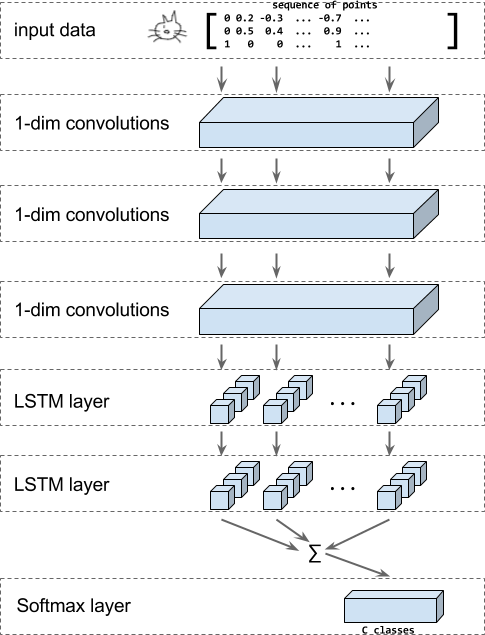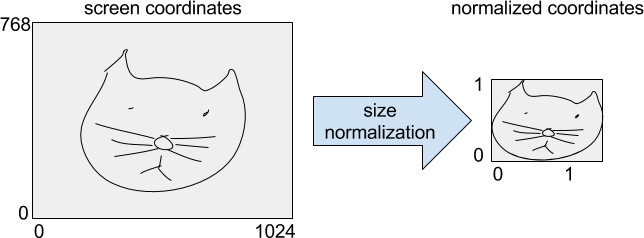Watch talks from the 2019 TensorFlow Dev Summit

# 涂鸦分类递归神经网络

Quick, Draw! 是一款游戏；在这个游戏中，玩家要接受一项挑战：绘制几个图形，看看计算机能否识别玩家绘制的是什么。

Quick, Draw! 的识别操作 由一个分类器执行，它接收用户输入（用 (x, y) 中的点笔画序列表示），然后识别用户尝试涂鸦的图形所属的类别。## 运行教程代码

1. 安装 TensorFlow（如果尚未安装的话）。
2. 下载教程代码
3. 点击此处下载数据TFRecord 格式），然后解压缩。如需详细了解如何获取原始 Quick, Draw! 数据以及如何将数据转换为 TFRecord 文件，请参阅下文。

4. 使用以下命令执行教程代码，以训练本教程中所述的基于 RNN 的模型。请务必调整路径，使其指向第 3 步中下载的解压缩数据。

  python train_model.py \
--training_data=rnn_tutorial_data/training.tfrecord-?????-of-????? \
--eval_data=rnn_tutorial_data/eval.tfrecord-?????-of-????? \
--classes_file=rnn_tutorial_data/training.tfrecord.classes


## 教程详情

### 可选：下载完整的 Quick Draw 数据

gsutil ls -r "gs://quickdraw_dataset/full/simplified/*"


gs://quickdraw_dataset/full/simplified/The Eiffel Tower.ndjson
gs://quickdraw_dataset/full/simplified/The Great Wall of China.ndjson
gs://quickdraw_dataset/full/simplified/The Mona Lisa.ndjson
gs://quickdraw_dataset/full/simplified/aircraft carrier.ndjson
...


mkdir rnn_tutorial_data
cd rnn_tutorial_data
gsutil -m cp "gs://quickdraw_dataset/full/simplified/*" .


### 可选：转换数据

   python create_dataset.py --ndjson_path rnn_tutorial_data \
--output_path rnn_tutorial_data


{"word":"cat",
"countrycode":"VE",
"timestamp":"2017-03-02 23:25:10.07453 UTC",
"recognized":true,
"key_id":"5201136883597312",
"drawing":[
[
[130,113,99,109,76,64,55,48,48,51,59,86,133,154,170,203,214,217,215,208,186,176,162,157,132],
[72,40,27,79,82,88,100,120,134,152,165,184,189,186,179,152,131,114,100,89,76,0,31,65,70]
],[
[76,28,7],
[136,128,128]
],[
[76,23,0],
[160,164,175]
],[
[87,52,37],
[175,191,204]
],[
[174,220,246,251],
[134,132,136,139]
],[
[175,255],
[147,168]
],[
[171,208,215],
[164,198,210]
],[
[130,110,108,111,130,139,139,119],
[129,134,137,144,148,144,136,130]
],[
[107,106],
[96,113]
]
]
}


def parse_line(ndjson_line):
"""Parse an ndjson line and return ink (as np array) and classname."""
class_name = sample["word"]
inkarray = sample["drawing"]
stroke_lengths = [len(stroke) for stroke in inkarray]
total_points = sum(stroke_lengths)
np_ink = np.zeros((total_points, 3), dtype=np.float32)
current_t = 0
for stroke in inkarray:
for i in [0, 1]:
np_ink[current_t:(current_t + len(stroke)), i] = stroke[i]
current_t += len(stroke)
np_ink[current_t - 1, 2] = 1  # stroke_end
# Preprocessing.
# 1. Size normalization.
lower = np.min(np_ink[:, 0:2], axis=0)
upper = np.max(np_ink[:, 0:2], axis=0)
scale = upper - lower
scale[scale == 0] = 1
np_ink[:, 0:2] = (np_ink[:, 0:2] - lower) / scale
# 2. Compute deltas.
np_ink = np_ink[1:, 0:2] - np_ink[0:-1, 0:2]
return np_ink, class_name### 定义模型

1. 将输入调整回原始形状，其中小批次通过填充达到其内容的最大长度。除了 ink 数据之外，我们还拥有每个样本的长度和目标类别。这可通过函数 _get_input_tensors 实现。

2. 将输入传递给 _add_conv_layers 中的一系列卷积层。

3. 将卷积的输出传递到 _add_rnn_layers 中的一系列双向 LSTM 层。最后，将每个时间步的输出相加，针对输入生成一个固定长度的紧凑嵌入。

4. _add_fc_layers 中使用 softmax 层对此嵌入进行分类。

inks, lengths, targets = _get_input_tensors(features, targets)
final_state = _add_rnn_layers(convolved, lengths)


### _get_input_tensors

shapes = features["shape"]
lengths = tf.squeeze(
tf.slice(shapes, begin=[0, 0], size=[params["batch_size"], 1]))
inks = tf.reshape(
tf.sparse_tensor_to_dense(features["ink"]),
[params["batch_size"], -1, 3])
if targets is not None:
targets = tf.squeeze(targets)


convolved = inks
for i in range(len(params.num_conv)):
convolved_input = convolved
if params.batch_norm:
convolved_input = tf.layers.batch_normalization(
convolved_input,
training=(mode == tf.estimator.ModeKeys.TRAIN))
# Add dropout layer if enabled and not first convolution layer.
if i > 0 and params.dropout:
convolved_input = tf.layers.dropout(
convolved_input,
rate=params.dropout,
training=(mode == tf.estimator.ModeKeys.TRAIN))
convolved = tf.layers.conv1d(
convolved_input,
filters=params.num_conv[i],
kernel_size=params.conv_len[i],
activation=None,
strides=1,
name="conv1d_%d" % i)
return convolved, lengths


outputs, _, _ = contrib_rnn.stack_bidirectional_dynamic_rnn(
cells_fw=[cell(params.num_nodes) for _ in range(params.num_layers)],
cells_bw=[cell(params.num_nodes) for _ in range(params.num_layers)],
inputs=convolved,
sequence_length=lengths,
dtype=tf.float32,
scope="rnn_classification")


mask = tf.tile(
[1, 1, tf.shape(outputs)])
zero_outside = tf.where(mask, outputs, tf.zeros_like(outputs))
outputs = tf.reduce_sum(zero_outside, axis=1)


tf.layers.dense(final_state, params.num_classes)


### 损失、预测和优化器

cross_entropy = tf.reduce_mean(
tf.nn.sparse_softmax_cross_entropy_with_logits(
labels=targets, logits=logits))
# Add the optimizer.
train_op = tf.contrib.layers.optimize_loss(
loss=cross_entropy,
global_step=tf.train.get_global_step(),
learning_rate=params.learning_rate,
# some gradient clipping stabilizes training in the beginning.
predictions = tf.argmax(logits, axis=1)
return model_fn_lib.ModelFnOps(
mode=mode,
predictions={"logits": logits,
"predictions": predictions},
loss=cross_entropy,
train_op=train_op,
eval_metric_ops={"accuracy": tf.metrics.accuracy(targets, predictions)})


### 训练和评估模型

  estimator = tf.estimator.Estimator(
model_fn=model_fn,
model_dir=output_dir,
config=config,
params=model_params)
# Train the model.
tf.contrib.learn.Experiment(
estimator=estimator,
train_input_fn=get_input_fn(
mode=tf.contrib.learn.ModeKeys.TRAIN,
tfrecord_pattern=FLAGS.training_data,
batch_size=FLAGS.batch_size),
train_steps=FLAGS.steps,
eval_input_fn=get_input_fn(
mode=tf.contrib.learn.ModeKeys.EVAL,
tfrecord_pattern=FLAGS.eval_data,
batch_size=FLAGS.batch_size),
min_eval_frequency=1000)